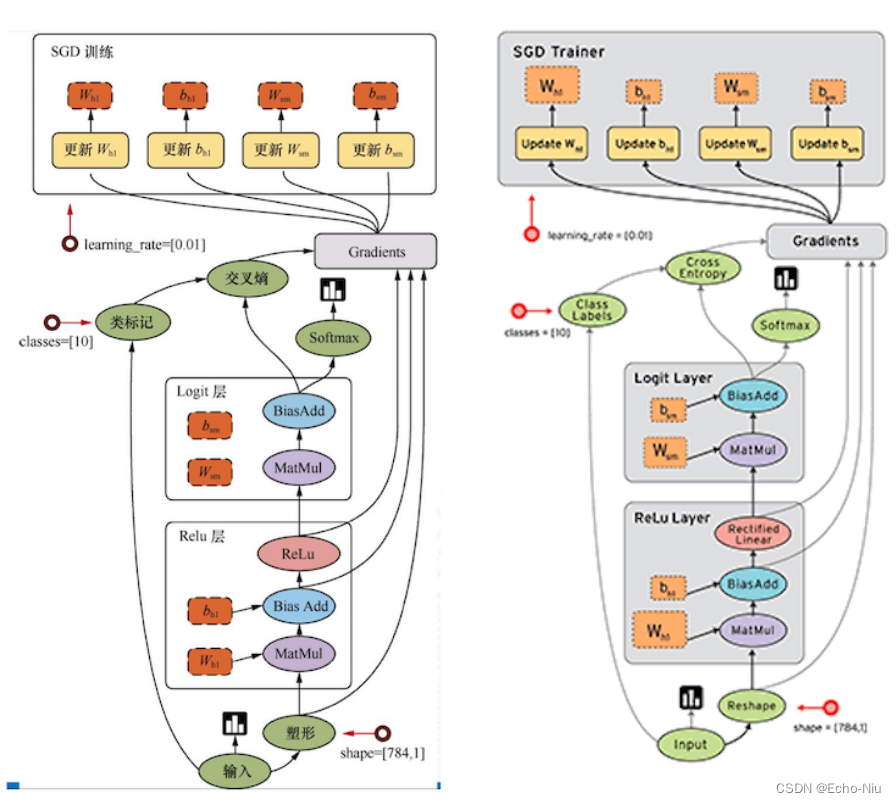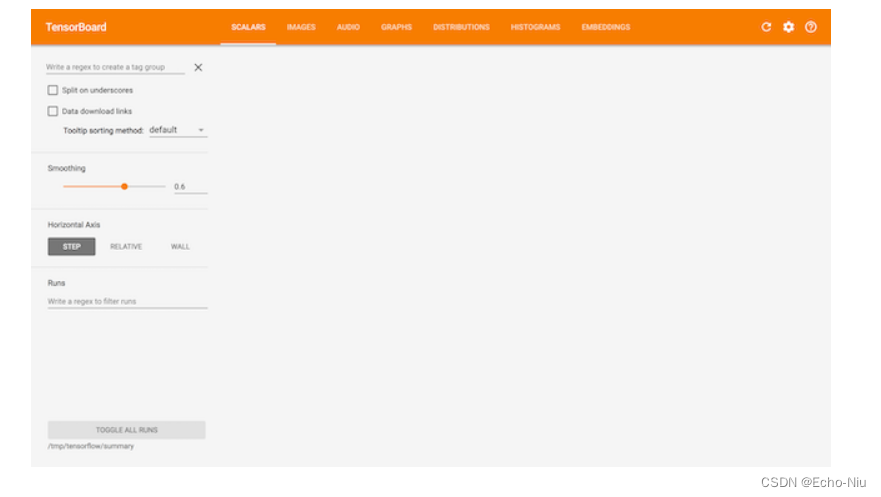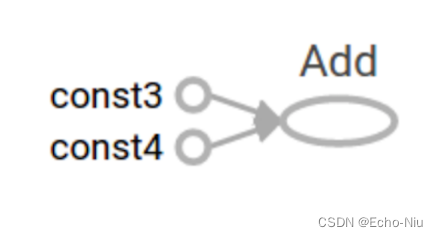# 2.1 TF数据流图

## 学习目标

• 目标
• 说明TensorFlow的数据流图结构
• 应用
• 内容预览
• 2.1.1 案例：TensorFlow实现一个加法运算
• 1 代码
• 2 TensorFlow结构分析
• 2.1.2 数据流图介绍

## 2.1.1 案例：TensorFlow实现一个加法运算

### 2.1.1.1 代码

``````def tensorflow_demo():
"""
通过简单案例来了解tensorflow的基础结构
:return: None
"""
# 一、原生python实现加法运算
a = 10
b = 20
c = a + b
print("原生Python实现加法运算方法1：\n", c)
return a + b
print("原生python实现加法运算方法2：\n", sum)

# 二、tensorflow实现加法运算
a_t = tf.constant(10)
b_t = tf.constant(20)
# 不提倡直接运用这种符号运算符进行计算
# 更常用tensorflow提供的函数进行计算
# c_t = a_t + b_t
print("tensorflow实现加法运算：\n", c_t)
# 如何让计算结果出现？
# 开启会话
with tf.Session() as sess:
sum_t = sess.run(c_t)
print("在sess当中的sum_t:\n", sum_t)

return None
``````

``````import os
os.environ['TF_CPP_MIN_LOG_LEVEL']='2'
``````

### 2.1.1.2 TensorFlow结构分析

TensorFlow 程序通常被组织成一个构建图阶段和一个执行图阶段。

• 图和会话 ：
• 图：这是 TensorFlow 将计算表示为指令之间的依赖关系的一种表示法
• 会话：TensorFlow 跨一个或多个本地或远程设备运行数据流图的机制
• 张量：TensorFlow 中的基本数据对象
• 节点：提供图当中执行的操作

## 2.1.2 数据流图介绍pyautogui

iTunes

TensorFlow是一个采用数据流图（data flow graphs），用于数值计算的开源框架。

dba

# 2.2 图与TensorBoard

## 学习目标

• 目标
• 说明图的基本使用
• 应用tf.Graph创建图、tf.get_default_graph获取默认图
• 知道开启TensorBoard过程
• 知道图当中op的名字以及命名空间
• 应用
• 内容预览
• 2.2.1 什么是图结构
• 2.2.2 图相关操作
• 1 默认图
• 2 创建图
• 2.2.3 TensorBoard:可视化学习
• 1 数据序列化-events文件
• 2 启动TensorBoard
• 2.2.4 OP
• 1 常见OP
• 2 指令名称

## 2.2.2 图相关操作

### 1 默认图

• 通过调用tf.get_default_graph()访问 ，要将操作添加到默认图形中，直接创建OP即可。
• op、sess都含有graph属性 ，默认都在一张图中
``````def graph_demo():
# 图的演示
a_t = tf.constant(10)
b_t = tf.constant(20)
# 不提倡直接运用这种符号运算符进行计算
# 更常用tensorflow提供的函数进行计算
# c_t = a_t + b_t
print("tensorflow实现加法运算：\n", c_t)

# 获取默认图
default_g = tf.get_default_graph()
print("获取默认图：\n", default_g)

# 数据的图属性
print("a_t的graph:\n", a_t.graph)
print("b_t的graph:\n", b_t.graph)
# 操作的图属性
print("c_t的graph:\n", c_t.graph)

# 开启会话
with tf.Session() as sess:
sum_t = sess.run(c_t)
print("在sess当中的sum_t:\n", sum_t)
# 会话的图属性
print("会话的图属性：\n", sess.graph)

return None
``````

### 2 创建图

• 可以通过tf.Graph()自定义创建图

os模块

• 如果要在这张图中创建OP，典型用法是使用tf.Graph.as_default()上下文管理器

windows 10

``````def graph_demo():
# 图的演示
a_t = tf.constant(10)
b_t = tf.constant(20)
# 不提倡直接运用这种符号运算符进行计算
# 更常用tensorflow提供的函数进行计算
# c_t = a_t + b_t
print("tensorflow实现加法运算：\n", c_t)

# 获取默认图
default_g = tf.get_default_graph()
print("获取默认图：\n", default_g)

# 数据的图属性
print("a_t的graph:\n", a_t.graph)
print("b_t的graph:\n", b_t.graph)
# 操作的图属性
print("c_t的graph:\n", c_t.graph)

# 自定义图
new_g = tf.Graph()
print("自定义图：\n", new_g)
# 在自定义图中去定义数据和操作
with new_g.as_default():
new_a = tf.constant(30)
new_b = tf.constant(40)

# 数据的图属性
print("new_a的graph:\n", new_a.graph)
print("new_b的graph:\n", new_b.graph)
# 操作的图属性
print("new_c的graph:\n", new_c.graph)

# 开启会话
with tf.Session() as sess:
sum_t = sess.run(c_t)
print("在sess当中的sum_t:\n", sum_t)
# 会话的图属性
print("会话的图属性：\n", sess.graph)
# 不同的图之间不能互相访问
# sum_new = sess.run(new_c)
# print("在sess当中的sum_new:\n", sum_new)

with tf.Session(graph=new_g) as sess2:
sum_new = sess2.run(new_c)
print("在sess2当中的sum_new:\n", sum_new)
print("会话的图属性：\n", sess2.graph)

# 很少会同时开启不同的图，一般用默认的图就够了
return None
``````

TensorFlow有一个亮点就是，我们能看到自己写的程序的可视化效果，这个功能就是Tensorboard。在这里我们先简单介绍一下其基本功能。

input

## 2.2.3 TensorBoard:可视化学习

TensorFlow 可用于训练大规模深度神经网络所需的计算，使用该工具涉及的计算往往复杂而深奥。为了更方便 TensorFlow 程序的理解、调试与优化，TensorFlow提供了TensorBoard 可视化工具。

armbianapp inventor

kcp

### 1 数据序列化-events文件

TensorBoard 通过读取 TensorFlow 的事件文件来运行，需要将数据生成一个序列化的 Summary protobuf 对象。

java进阶

``````# 返回filewriter,写入事件文件到指定目录(最好用绝对路径)，以提供给tensorboard使用
tf.summary.FileWriter('./tmp/summary/test/', graph=sess.graph)
``````

``````events.out.tfevents.{timestamp}.{hostname}
``````

### 2 启动TensorBoard

``````tensorboard  --logdir="./tmp/tensorflow/summary/test/"
``````

Monkey测试https

## 2.2.4 OP

### 2.2.4.1 常见OP

``````# 实现一个加法运算
con_a = tf.constant(3.0)
con_b = tf.constant(4.0)

print("打印con_a：\n", con_a)
print("打印con_b：\n", con_b)
print("打印sum_c：\n", sum_c)
``````

``````打印con_a：
Tensor("Const:0", shape=(), dtype=float32)

Tensor("Const_1:0", shape=(), dtype=float32)

``````

• "<OP_NAME>" 是生成该张量的指令的名称
• "<i>" 是一个整数，它表示该张量在指令的输出中的索引

### 2.2.4.2 指令名称

tf.Graph对象为其包含的 tf.Operation对象定义的一个命名空间。TensorFlow 会自动为图中的每个指令选择一个唯一名称，用户也可以指定描述性名称，使程序阅读起来更轻松。我们可以以以下方式改写指令名称

• 每个创建新的 tf.Operation 或返回新的 tf.Tensor 的 API 函数可以接受可选的 name 参数。

• 当修改好之后，我们在Tensorboard显示的名字也会被修改
``````a = tf.constant(3.0, name="a")
b = tf.constant(4.0, name="b" )
``````